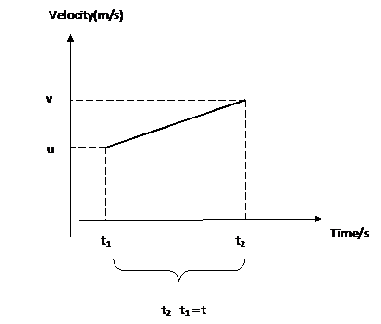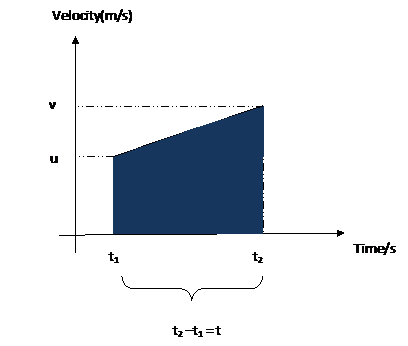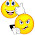Custom Search
If you want to discuss more on this or other issues related to Physics, feel free to leave a message on my Facebook page.

## Sunday, January 16, 2011

### Second equation of motion

We have seen in a previous post the first equation of motion.

Today we are going to see the second equation of motion.

You would remember that the first equation dealt with acceleration, time taken, initial velocity and final velocity.

So how do we derive the second equation of motion?

Let us take an object that starts from an initial velocity u and then accelerates during a time t until it reaches a final velocity v.

Now during the time the object was accelerating the object has moved a distance s. In the second equation of motion we will try to derive the equation to calculate this distance s.

Fig 2 below shows what is happening to the object.Fig 1

Now you would remember that the area under a velocity-time graph is the distance travelled by the object. Hence we are going to calculate the area under the graph as indicated by the shaded area below.Fig 2

Now if s the distance travelled is the area under a velocity-time graph then we can say that

Area under graph = 1/2( v + u)*t

hence s = 1/2( v + u)*t

Now if you can remember the first equation of motion is

v = u +at

Hence if we replace v = u +at into s = 1/2( v + u)*t we get

s = 1/2( v + u)*t

s = 1/2( [u +at] + u)*t

s = 1/2( u + at + u)*t

s = 1/2(2 u + at )*t

s =1/2(2ut +at2)

S = ut + 1/2at2

Hence as you can see above the second equation of motion is

S = ut + 1/2at2

We are now going to look at two examples where the second equation of motion can be used.

Example 1

A bus starts from rest and accelerates at a rate of 2.5 m/s2 for a time of 50 s. Determine the distance travelled by the bus during the acceleration phase.

Now let us identify the different variables involved.

u the initial speed = 0 m/s

t time taken = 50 s

a acceleration = 2.5 m/s2

s distance travelled = ???

If we want calculate s the distance travelled we will have to use the second equation of motion

S = ut + 1/2at2

Substituting the different values we get

S = ut + 1/2at2

= o*50 + 0.5* 2.5*502

= 0 + 1.25*2500

=3125 m

Example 2

An aero plane travelling at a speed of 300 m/s lands on a track 2000 m long and decelerates for a time of 25 s until it comes to rest. Calculate the deceleration needed to stop the plane.

We must now identify the different variables involved as in the first example.

initial speed u = 300 m/s

time taken t = 25 s

Distance travelled s = 2000 m

acceleration a = ????

If we want calculate the acceleration a we will have to use the second equation of motion

S = ut + 1/2at2

and make a the subject of formula.

s – ut = 1/2at2

a =2(s - ut)/t2

= 2(2000 – 300*25)/252

= –8.8 m/s2

As you can see using the second equation of motion is not that difficult. Should you enter into any difficulties just post the question into the comment section.

1.hey thanks for your help...you have EXACTLY the same type of problem that was on my worksheet that I needed help with. Thanks a lot and keep working on the blog!

2.Hey, I had a great time reading your website. Do you have an email address that I can contact you on? Thank you and hope to hear from you soon.

Regards,

Joel
JHouston791 gmail.com

3.good notes. thanks